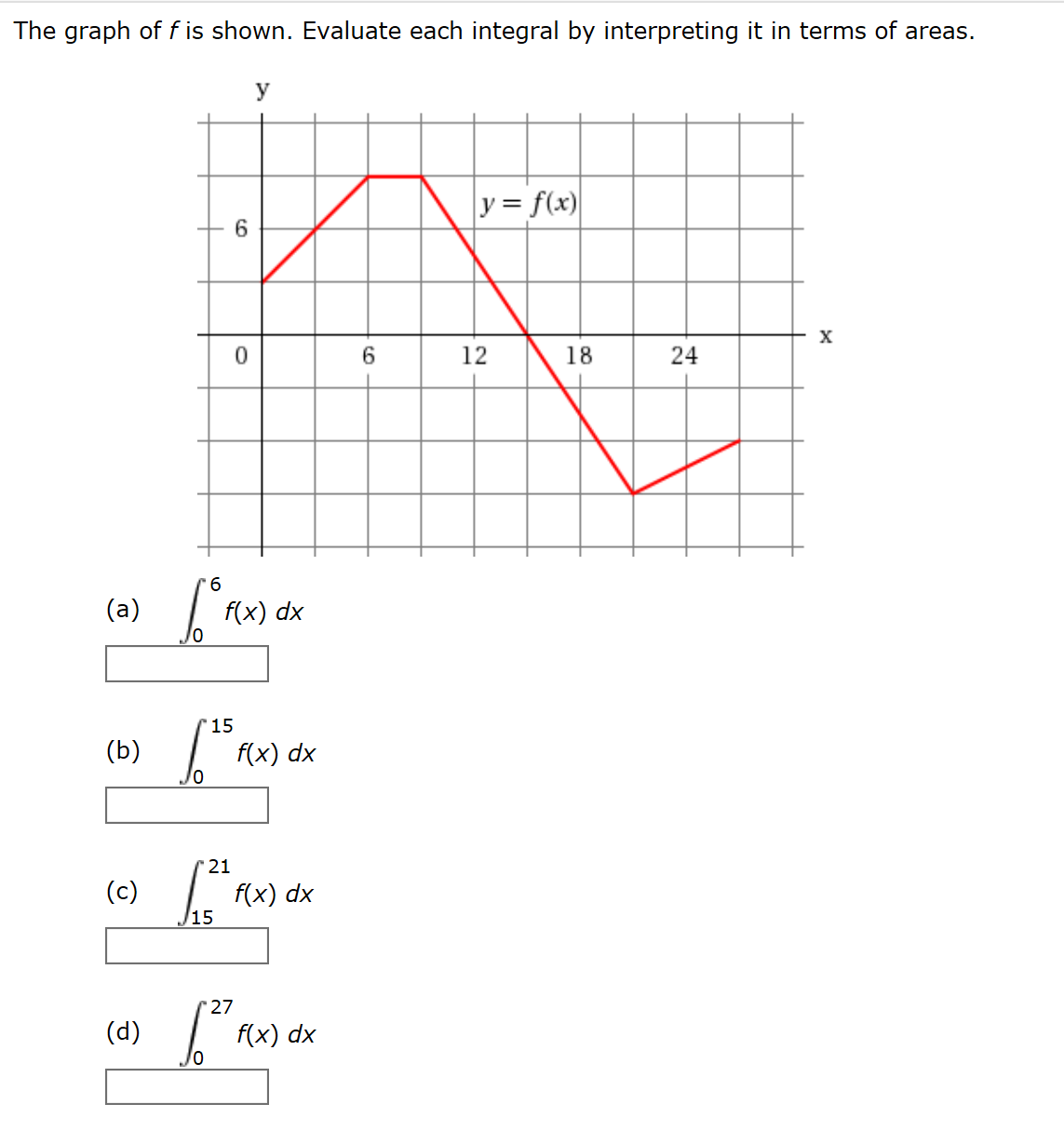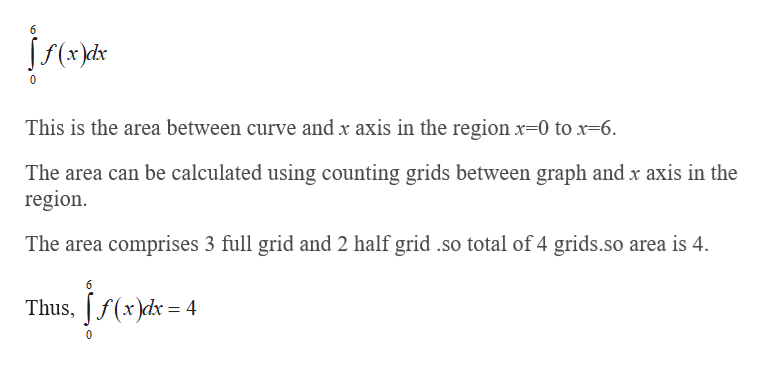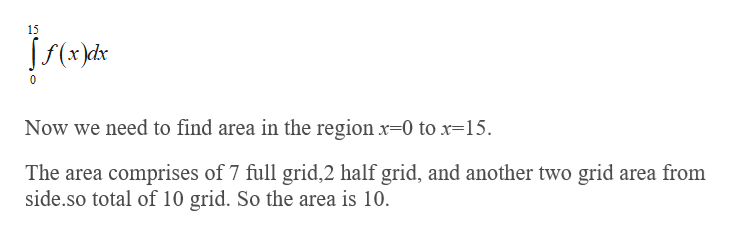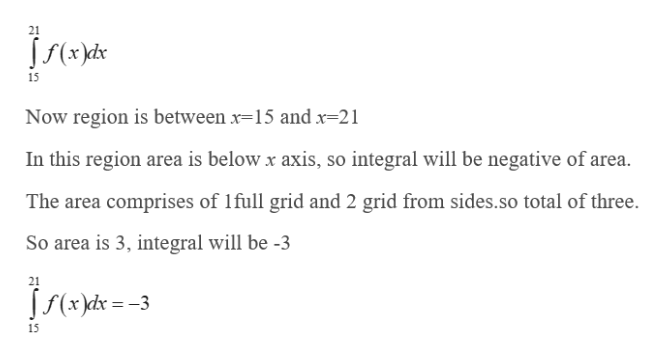# The graph off is shown. Evaluate each integral by interpreting it in terms of areas.Уyf(x)х018(a)f(x) dx(b)f(x) dx(c)f(x) dx15(d)f(x) dx2412

Question
16 viewshelp_outlineImage TranscriptioncloseThe graph off is shown. Evaluate each integral by interpreting it in terms of areas. У yf(x) х 0 18 (a) f(x) dx (b) f(x) dx (c) f(x) dx 15 (d) f(x) dx 24 12 fullscreen
check_circle

Step 1

By integration with respect to x axis it means that find area between the curve and x axis.

If curve is below the line, then integration result will be negative of area between curve and x axis.

(a) Evaluate the value of the given integral.help_outlineImage Transcriptionclose6 This is the area between curve and x axis in the region x-0 to x-6 The area can be calculated using counting grids between graph and x axis in the region. The area comprises 3 full grid and 2 half grid so total of 4 grids.so area is 4. Thus, f(x)dx 4 0 fullscreen
Step 2

(b) Evaluate the value of the given integral.help_outlineImage Transcriptionclose15 Now we need to find area in the region x-0 to x-15. The area comprises of 7 full grid,2 half grid, and another two grid area from side.so total of 10 grid. So the area is 10. fullscreen
Step 3

(c) Evaluate the value of ...help_outlineImage Transcriptionclose21 15 Now region is between x-15 and x-21 In this region area is below x axis, so integral will be negative of area. The area comprises of 1full grid and 2 grid from sides.so total of three. So area is 3, integral will be -3 21 Jf(xd3 15 fullscreen

### Want to see the full answer?

See Solution

#### Want to see this answer and more?

Solutions are written by subject experts who are available 24/7. Questions are typically answered within 1 hour.*

See Solution
*Response times may vary by subject and question.
Tagged in

### Other